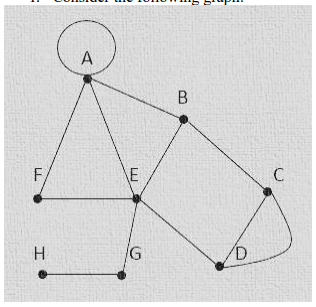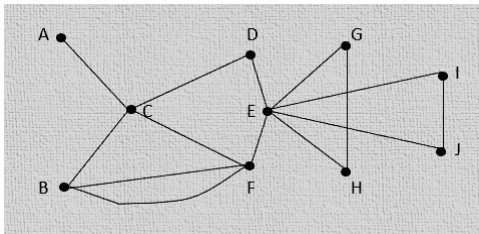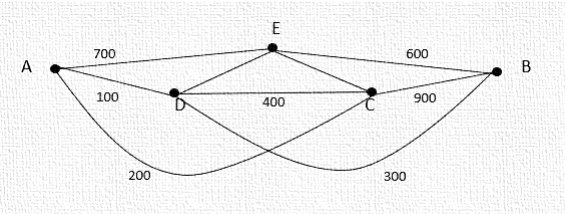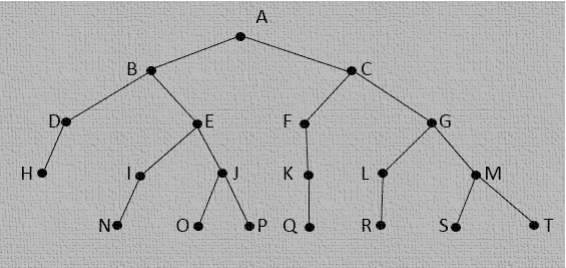# Consider the following graph

25.00 \$

Categories: ,
Click Category Button to View Your Next Assignment | Homework

You'll get a: . ` docx` file solution, download link after Payment

## Description

Assignment Description
Complete the following in a paper of 1–2 pages:
1. Consider the following graph:a. Complete this table by finding the degree of each vertex, and identify whether it is even
or odd:
Vertex Degree Even/Odd
A
B
C
D
E
F
G
H
b. What is the order of the graph?
c. Construct the 10 x 10 adjacency matrix for the graph.
2. The graph below illustrates a switching network. The weights represent the delay times, in
nanoseconds, travelled by a data packet between destinations, represented by the vertices.
a. Complete the following table by finding the shortest distance and the path for that distance
from vertex A to the other vertices:Vertex Shortest Distance from A Path from A
B
C
D
E
F
G
H
b. What is the shortest distance between A and J and the path for that distance?
3. The following graph represents a portion of the subway system of a city. The vertices on the
graph correspond to subway stations, and the edges correspond to the rails. Your job is to write a
program for a cleaning car to efficiently clean this portion of the subway system.a. Using Euler’s theorem, explain why it is possible to pass through all of the stations by tra
versing every rail only once.
b. Using Fleury’s algorithm, provide an optimal path to clean all the rails by passing throug
h them only once.
c. Is it possible to find an optimal path described in question 3-b that starts on any station?
d. Is it possible to find an optimal path described in question 3-b that starts and ends at the
same station? Explain why or why not.
4.
A network engineer lives in City A, and his job is to inspect his company’s servers in various citi
es. The graph below shows the cost (in U.S. dollars) of travelling between each city that he has
to visit.a. Find a Hamiltonian path in the graph.
b. Find a Hamiltonian circuit that will allow the engineer to inspect all of the servers. How much
will the cost be for his trips?
c. Is there another Hamiltonian circuit that will allow the engineer to inspect all of the servers
other than your answer in question 4-b? If so, calculate the cost.
5. Consider the following binary tree:a. What is the height of the tree?
b. What is the height of vertex H?
c. Write the preorder traversal representation of the tree.
d. Write the array representation of the tree by completing the following table:
Vertex Left Child Right Child
A
B
C
D
E
G
H
I
J
K
L
M
N
O
P
Q
R
S
T
Please cite references for this assignment in APA format.
Thank you for your help with this assignment.
graciousbc